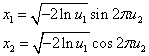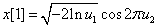ID 772987
Date 12/04/2020
Public

## Gaussian (VSL_RNG_METHOD_GAUSSIAN_BOXMULLER2)

Random number generator of normal (Gaussian) distribution with parameters a and s. You can produce a successive pair of the random numbers x1, x2 of the standard normal distribution according to the formulawhere u1, u2 are a pair of successive random numbers uniformly distributed over the interval (0, 1). For details, see [Box58].

The normal distribution with the parameters a and s is transformed to the random number y by scaling and the shift y = sx+a.

You can safely call this VS method even when the random numbers are generated in blocks with the size aliquant to 2.

For example, you use the VSL_METHOD_DGAUSSIAN_BOXMULLER2 method to generate a pair of random numbers of the standard normal distribution.

### Option 1

Single call of method VSL_METHOD_DGAUSSIAN_BOXMULLER2 with the vector length equal to 2:

...
double x;
...
vdRngGaussian(VSL_RNG_METHOD_GAUSSIAN_BOXMULLER2, stream, 2, x, 0.0, 1.0);
...


In this case, you generate the random numbers x, x by the formula:### Option 2

Double call of the method VSL_METHOD_DGAUSSIAN_BOXMULLER2 with the vector length equal to 1:

...
double x;
...
vdRngGaussian(VSL_RNG_METHOD_GAUSSIAN_BOXMULLER2, stream, 1, &x, 0.0, 1.0);
vdRngGaussian(VSL_RNG_METHOD_GAUSSIAN_BOXMULLER2, stream, 1, &x, 0.0, 1.0);
...


At the first call of vdRngGaussian you produce the random number x by the formula:At the second call of vdRngGaussian the vector length, over which you initially called the function to generate the random stream, is recognized as odd (equal to 1 in this case). Then the random number x is generated by the formula:and not by the formula:See Intel® oneAPI Math Kernel Library Vector Statistics Random Number Generator Performance Data for test results summary.